# Comparing Accuracy Rate of Classification Algorithms Using PythonPhoto by Jorge Franganillo on Unsplash

As discussed in the previous article via this link, we want to make a supervised learning classification to predict the churn rate from the telecommunication dataset. Before we go any further, the source codes and dataset can be downloaded from this Github.

Supervised learning is grouped into regression and classification. Regression is an analytical technique to identify a relationship between two or more variables that aim to find a function by modeling data to minimize the error or difference between the predicted value and the actual value. This technique is used to predict continuous values.

In contrast to regression, classification is a technique for classifying or categorizing several unlabeled items into a set of discrete classes. This technique tries to learn the connection between a set of feature variables and target variables. There are some classification algorithms out there, but now we will try to compare the accuracy rate using these algorithms listed below:

• Decision Tree
• Random Forest
• K-Nearest Neighbors (KNN)
• Naive Bayes
• Support Vector Machine (SVM)

1. Decision Tree

Decision Tree is a method for estimating the discrete value target function, where a decision tree represents the learning function. This method is used to classify a sample of data whose class is not yet known into existing classes.

The basic structure is shaped like a tree structure where each internal node states a test of an attribute, each branch states the test’s output, and the leaf node states the classes or class distribution. The topmost node is referred to as the root node.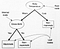Image 1. Root and leaf relation in Decision Tree (Source: link)

Now, let us jump to the code of the Decision Tree below. First, we define the selected non-target variables based on the method in this link. Then, we also need to define the variable target.

After that, import function train_test_split from Sklearn model selection for splitting data arrays into training and testing. Set the testing size of 20% dataset, which means that the training dataset is 80%.

After finish the splitting process, we move to do feature scaling (normalization) to make numerical data on the dataset have the same range of values (scale). No longer one data variable dominates the other data variables. Again, we divide this process into training and testing.

We will use xtrain and xtest from the code above to implement the decision tree’s core process. The algorithm in this method uses an entropy concept, which is implemented to measure how informative or useful a node is. The node will be applied to calculate gain information, measuring the effectiveness of an attribute in classifying data, and determining the order of attributes where the attribute with the largest information gain value is selected.

Then we will make a confusion matrix, performance measurement for machine learning classification problems where the output can be two or more classes. The confusion matrix is a matrix with four different combinations of predicted and actual values. Four terms represent the classification process results in the confusion matrix: True Positive, True Negative, False Positive, and False Negative. To understand more about the confusion matrix, let’s check this explanation below and the visualization in Image 2.

• True Positive (TP): when we predict positive and it turns out true. Ex.: we predict that cows are mammals, and they are.
• True Negative (TN): when we predict negative and it turns out true. Ex.: we predict that birds are not mammals, and they are not.
• False Positive (FP): when we predict positive and it turns out false. Ex.: we predict that birds are mammals, but they are not.
• False Negative (FN): when we predict negative and it turns out false. Ex.: we predict that cows are not mammals, but they are.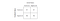Image 2. Structures of predicted and actual values (Source: link)

Since we have understood the theory, let us start to implement those explanations above into the code below:Image 3. The result of confusion matrix after done with Decision Tree

As we see from Image 3, we get 823 of TP, 202 of FP, 202 of FN, and 172 of TN from the Decision Tree model we have made. To understand more about the confusion matrix result, we will run this code below to get the value of Precision, Recall, F1-score, and Accuracy.

Before we see the result, I will explain each component that will show on the report:

• Precision: describes the accuracy between the requested data and the prediction results provided by the model.

Precision = (TP) / (TP + FP)

• Recall (sensitivity): describes the success of the model in recovering information.

Recall = TP / (TP + FN)

• F1-Score: describes the weighted comparison of the weighted average of precision and recall as another consideration option if the calculation of accuracy uses a dataset where the number of False Negatives and False Positives is not as close (asymmetric).

F-1 Score = (2 * Recall * Precision) / (Recall + Precision)

• Accuracy: describes how accurate the model is correctly classified.

Accuracy = (TP+TN) / (TP+FP+FN+TN)

Now, we will see the result of the classification report shown in Image 4.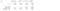Image 4. Classification report of Decision Tree

The result above shows that our model from the Decision Tree method has 71% accuracy, with the same percentage of weighted average precision and recall. To understand more about how to read the report, you can go to this website and this one.

2. Random Forest

The Random Forest method is one of the methods in the Decision Tree, the combination of each tree is combined into one model, as shown in Image 5. Random Forest depends on a random vector value with the same distribution in all trees in which each decision tree has the maximum depth, this is what makes it different from the decision tree that is built on an entire dataset by using all the features.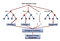Image 5. The visualization of Random Forest (Source: link)

To get the Random Forest result, we can use all steps on implementing Decision Tree codes except for step 6. We will use codes in steps 1–5 and 7–8, but rewrite the code in step 6 with this code below. The Confusion Matrix results from Random Forest shown in Image 6 and Image 7 describe the classification report.Image 6. The result of confusion matrix after done with Random Forest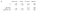Image 7. Classification report of Random Forest

The result of Random Forest algorithm above shows that our model has 74% of accuracy with 73% of weighted average precision and 74% of recall. As I mentioned before, we will use the F1-Score result as another option if the accuracy calculation uses a dataset where the number of FN and FP is asymmetric. We will use an accuracy percentage of 73% (F1-Score weighted average).

3. K-Nearest Neighbors (KNN)

KNN is a classification method for a set of data based on learning previously classified data. The newly classified query results are based on the majority of the proximity of existing categories in the k-nearest neighbor category.

The steps of K-Nearest Neighbors will be in the following order:

1. Specify the parameter k (number of closest neighbors).
2. Calculate the square of the object’s euclidean distance against the given training data.
3. Sort the results number 2 in ascending order (sequentially from high to low values)
4. Collect the classification of nearest-neighbor based on k-value
5. By using the most majority nearest neighbor category, the object category can be predicted.

To understand more about KNN, we can see the visualization concept of this algorithm in Image 8.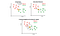Image 8. Visual concept of K-Nearest Neighbors (Source: link)

Now, we will implement the KNN method into codes. First, follow the code steps of the Decision Tree algorithm from step 1 to step 4. Then write these codes below before jump to code step 7–8 to get the confusion matrix and classification report. The final result will be like Image 9 for the confusion matrix and Image 10 for the classification report.Image 9. The result of confusion matrix after done with K-Nearest Neighbors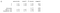Image 10. Classification report of K-Nearest Neighbors

Based on the results above, we can summarise that the accuracy of the K-Nearest Neighbors algorithm for the dataset is 76% (it should be 77%, but since the number of FN and FP is asymmetric, we will use F1-Score weighted average). Other than that, weighted average precision and recall are 75% and 77%, respectively.

4. Naive Bayes

This method aims to predict future opportunities based on previous experience, based on Bayes’s Theorem. This Naïve Bayes Classifier’s main characteristic is a firm assumption (naive) of each condition/event’s independence.
To understand more on how Naive Bayes works, we first need to understand Bayes’s Theorem introduced by Thomas Bayes. Bayes Theorem is a theorem to relate prior (initial belief) to posterior (new belief) after a new observation or evidence based on a certain probability. This below is the standard expression of Bayes’s Theorem:

P(A|B) = P(B|A) x P(A) / P(B)

For example, the probability of someone getting Covid-19 (A) when they are having influenza (B) can be written P (A | B). The implication of the theorem is often used to perform reverse probability calculations. If we find it challenging to determine P (B | A), then calculate P (A | B). This approach means that if we have trouble calculating someone getting Covid-19, then start by calculating the chance of someone having influenza.

Another example that may happen in real-life situations is predicting the amount of household electricity usage using historical data of factors such as the number of people in a building, building area, monthly income, and electrical power. Then link those variables with historical electricity usage to predicts future opportunities.

Lets we jump into the algorithm implementation. First, write all the codes from Decision Tree step 1–5, then change the sixth step with this code below before reuse the code from Decision Tree step 7–8 to get the confusion matrix (Image 11) and classification report (Image 12).Image 11. The result of confusion matrix after done with Naive Bayes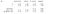Image 12. Classification report of Naive Bayes

Since the FN and FP number are asymmetric (Image 11), we will use the F1-Score weighted average accuracy percentage, 76%. The weighted average precision number is 76%, while the recall is 77%.

5. Support Vector Machine (SVM)

Support Vector Machine is simply described as an attempt to find the best hyperplane, which functions as a separator of two data classes in the input space. This technique is used to obtain the optimal separator function (hyperplane) for separating observations with different target variable values.

To determine the decision boundary, which is a linear or hyperplane model with weight and bias parameters, SVM uses the margin concept that is defined as the closest distance between the decision boundary and any training data. We can obtain a specific decision boundary by maximizing the margin.

Image 13 gives us a more precise visualization of how the Support Vector Machine method works.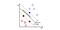Image 13. The concept of Support Vector Machine (Source: link)

This way, we can implement the explanation above into the codes below. As we have done before the other methods, let’s copy the code of Decision Tree step 1–4. Then continue the code with this particular SVM code below. After that, write the code again from Decision Tree step 7–8.

After running the code from Decision Tree step 7–8, we will get the result below on Image 14 for the confusion matrix and 15 for the classification report.Image 14. The result of confusion matrix after done with Support Vector Machine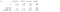Image 15. Classification report of Support Vector Machine

This method shows a different confusion matrix than the other methods in that both number of False Positive and True Negative are false. For some reason, this is a classic problem in machine learning called an unbalanced problem. It is a matter of the number of samples from one class being far higher than the number of samples from another class. However, it can affect the accuracy of the model, so we need to tackle this issue in another chance.

This method gives us an accuracy number of 62% (based on the F1-Score) and 54% of precision weighted average, while recall percentage has the highest number among the others with 73%.

CONCLUSION

Based on the practice that has been conducted, summarised in the table of Image 16, methods that obtain the highest accuracy percentage to predict churn rate for telco dataset are KNN and Naive Bayes. The model of SVM is the least accurate model compared to the others because of the unbalanced problem. Among all methods, only the Decision Tree algorithm model has the same percentage in its accuracy, precision, and recall, which probably means that FP=FN makes those three metrics have identical values.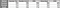Image 16. The comparison of Classification Methods results

Finally, the series of steps toward Machine Learning Classification has been completed. If you want to recall the article about data cleaning, click this link. After cleaning the data, you can continue to read and implement the codes about data encoding and feature selection through this link.

Thank you for reading this article, see you on the next writing!